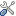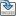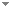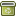Programming Planck units from a virtual electron; a Simulation Hypothesis

 Authors Abstract The simulation hypothesis proposes that all of reality is an artificial simulation. In this article I describe a simulation model that derives Planck level units as geometrical forms from a virtual (dimensionless) electron formula $f_e$ that is constructed from 2 unit-less mathematical constants; the fine structure constant $\alpha$ and $\Omega$ = 2.00713494... ($f_e = 4\pi^2r^3, r = 2^6 3 \pi^2 \alpha \Omega^5$). The mass, space, time, charge units are embedded in $f_e$ according to these ratio; ${M^9T^{11}/L^{15}} = (AL)^3/T$ (units = 1), giving mass M=1, time T=$2\pi$, length L=$2\pi^2\Omega^2$, ampere A = $(4\pi \Omega)^3/\alpha$. We can thus for example create as much mass M as we wish but with the proviso that we create an equivalent space L and time T to balance the above. The 5 SI units $kg, m, s, A, K$ are derived from a single unit u = sqrt(velocity/mass) that also defines the relationships between the SI units; kg = $u^{15}$, m = $u^{-13}$, s = $u^{-30}$, A = $u^{3}$, $k_B = u^{29}$. To convert MLTA from the above $\alpha, \Omega$ geometries to their respective SI Planck unit numerical values (and thus solve the dimensioned physical constants $G, h, e, c, m_e, k_B$) requires an additional 2 unit-dependent scalars. Results are consistent with CODATA 2014. The rationale for the virtual electron was derived using the sqrt of momentum P and a black-hole electron model as a function of magnetic-monopoles AL (ampere-meters) and time T. Keywords Simulation Hypothesis  Simulation Universe  Virtual electron  Black hole electron  Planck unit  fine structure constant  sqrt Planck momentum  magnetic monopole Categories (categorize this paper) OptionsEdit this recordMark as duplicateExport citationFind it on ScholarRequest removal from indexRevision history

PhilArchive copy

Upload a copy of this paper     Check publisher's policy     Papers currently archived: 65,657

Setup an account with your affiliations in order to access resources via your University's proxy server
Configure custom proxy (use this if your affiliation does not provide a proxy)

References found in this work BETA

No references found.